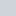## Cymath Math Problem Solver 2.27 Plus APK(No Ratings Yet)Loading...
55 DownloadsWith millions of users worldwide Cymath.com, the Cymath math problem-solving app uses the same math engine while allowing you to solve problems on your travels! Just enter a problem with your math homework, and let Cymath solve for you step by step! We provide algebra, as well as calculus help.
Our math problem solving is designed to help you do your math homework. In addition to homework answers, we also show you how to get there step by step. Let Cymath help you do your math homework today!

Supported Android
{4.2 and UP}
Supported Android Version:-
Jelly Bean (4.2–4.3.1) - KitKat (4.4–4.4.4) - Lollipop (5.0–5.0.2) - Marshmallow (6.0 - 6.0.1) - Nougat (7.0 – 7.1.1) - Oreo (8.0-8.1)

# Download Cymath Math Problem Solver 2.27 Plus APK For Android

Cymath Math Problem Solver Plus v2.27.apk